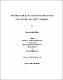## Thermal modelling of a natural-convection-cooled, oil-immersed distribution transformer##### Date
1997-05-01T00:00:00Z
##### Authors
Marko, Robert Michael
##### Abstract
A computer code was developed to implement a numerical model that predicts the temperature field in and around the core and windings of a natural-convection-cooled distribution transformer. The computer code also predicts the two-dimensional flow of transformer oil around the core and windings of a transformer. A key feature of the presented model is its detailed treatment of the geometry in two dimensions. The model requires detailed information about the transformer geometry and materials. A solver was developed based on the additive correction multigrid solver algorithm. The model consisted of a set of differential equations expressing conservation of mass, momentum, and energy, over a Cartesian domain. The fluid was assumed to have Newtonian and laminar flow characteristics. The energy equation was modified to allow for conjugate heat transfer between a solid and the surrounding fluid. A method for modelling the windings as a homogeneous material was also introduced. The transport equations were discretized using a finite volume approach, and the pressure-velocity coupling was handled using the SIMPLEC algorithm. Tests were performed to validate the computer code. The code was compared against another transformer model to show that it could model general transient temperature trends. A parametric study was conducted to show the effect of two ambient conditions, and the effect of having oil viscosity modelled as a function of temperature, on the solutions. The effect of modelling the core and windings as a homogeneous region was also explored. The code was tested against the ANSI loading guides for overloads. Although maximum hotspot temperatures by the code were relatively close to those predicted by the loading guides, the maximum oil temperature predicted was much lower than ANSI. The difference between the maximum temperatures was decreased when variable viscosity was employed. (Abstract short ned by UMI.)